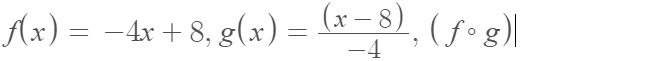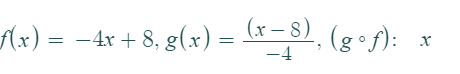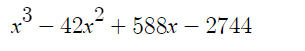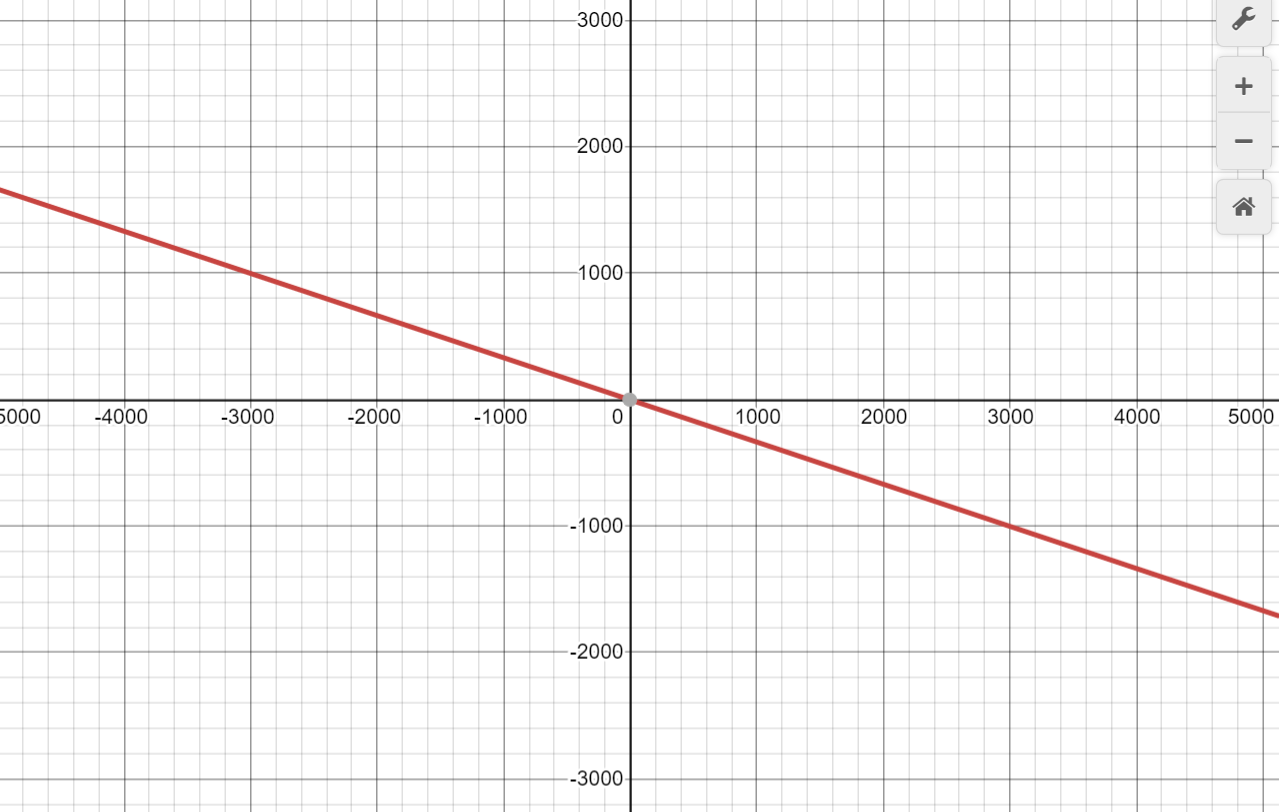### Still have math questions?

Algebra
Question16. Given the following functions, use function composition to determine if $$f ( x )$$ and $$g ( x )$$ are inverse functions.

$$f ( x ) = - 4 x + 8$$ and $$g ( x ) = \frac { x - 8 } { - 4 }$$

$$( f \circ g ) ( x ) =$$       $$f$$$$( g \circ f ) ( x ) =$$

Thus $$g ( x )$$ is not $$/$$ is (circle one $$)$$ the inverse function of

17.Find the inverse function of $$f ( x ) = 14 + \sqrt[ 3 ] { x }$$ and specify the domain for $$f ^ { - 1 } ( x )$$ using interval notation.

$$f ^ { - 1 } ( x ) =$$

Domain of $$f ^ { - 1 } ( x ) :$$

18.Given the graph of $$f ( x ) = - 3 x - 9 ,$$ sketch the graph of its inverse $$f ^ { - 1 } ( x )$$

16)17)$$Domain\space of\space f^{- 1}= - \infty\space to\space \infty$$

18)Solution
View full explanation on CameraMath App.